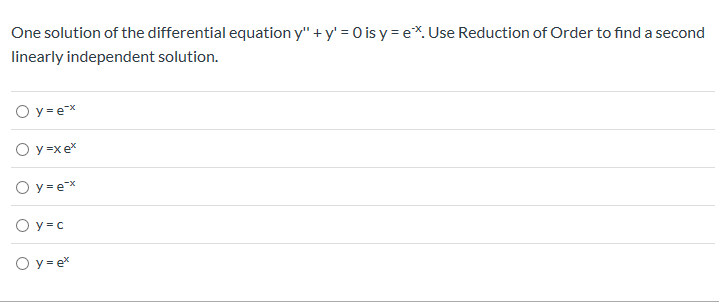# One solution of the differential equation y" +y' = O is y = e. Use Reduction of Order to find a secondlinearly independent solution.O y eОу-хе*Оу-ехO y cОу-ех

Question

Helphelp_outlineImage TranscriptioncloseOne solution of the differential equation y" +y' = O is y = e. Use Reduction of Order to find a second linearly independent solution. O y e Оу-хе* Оу-ех O y c Оу-ех fullscreen
check_circleExpert Solution
Step 1

In such problems ie second order linear differential equations when we are given one solution,y1 we assume the second solution to be of the form, y2=vy1 and substitute y2 in the given ode and reduce order of the differential equation by using the fact that y1 is a solution.

Compute:y2,y2’’

Step 2

Substitute y2,y2’’ in given differential equation

Step 3

Let u=v’ we get a first order dif...

### Want to see the full answer?

See Solution

#### Want to see this answer and more?

Solutions are written by subject experts who are available 24/7. Questions are typically answered within 1 hour*

See Solution
*Response times may vary by subject and question
Tagged in

### Calculus